# PSAT Math : How to find the square of an integer

## Example Questions

### Example Question #21 : Basic Squaring / Square Roots

Simplify.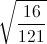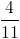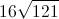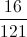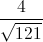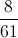Explanation:Take the square root of both the top and bottom terms.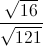Simplify.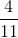### Example Question #1 : How To Find The Square Of An Integer

The square root of 5184 is:

71

72

73

74

70

72

Explanation:

The easiest way to narrow down a square root from a list is to look at the last number on the squared number – in this case 4 – and compare it to the last number of the answer.

70 * 70 will equal XXX0

71 * 71 will equal XXX1

72 * 72  will equal XXX4

73 * 73 will equal XXX9

74 * 74  will equal XXX(1)6

Therefore 72 is the answer.  Check by multiplying it out.

### Example Question #1 : Other Squaring / Square Roots

If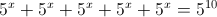, what is the value of x?

10

1

2

5

9

9

Explanation: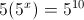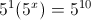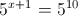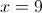### Example Question #2 : How To Find The Square Of An Integer

If x and y are integers and xy + y2 is even, which of the following statements must be true?

I. 3y is odd

II. y/2 is an integer

III. xy is even

I, II, & III

II only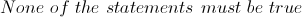I & II

I onlyExplanation:

In order for the original statement to be true, the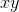andterms must be either both odd or both even. Looking at each of the statements individually,

I. States that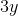is odd, but only odd values multiplied by 3 are odd. Ifwas an even number, the result would be even. Butcan be either odd or even, depending on whatequals. Thus this statement COULD be true but does not HAVE to be true.

II. States that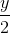is an integer, and since only even numbers are cleanly divided by 2 (odd values result in a fraction) this ensures thatis even. However,can also be odd, so this is a statement that COULD be true but does not HAVE to be true.

III. For exponents, only the base value determines whether it is even or odd - it does not indicate the value of y at all. Only even numbers raised to any power are even, thus, this ensures thatis even. Butcan be odd as well, so this statement COULD be true but does not HAVE to be true.

An example of two integers that will work violate conditions II and III isand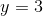.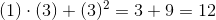, and even number.is not an integer.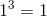is not even.

Furthermore, any combination of 2 even integers will make the original statement true, and violate the Statement I.

### Example Question #3 : How To Find The Square Of An Integer

How many integers from 20 to 80, inclusive, are NOT the square of another integer?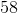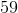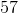Explanation:

First list all the integers between 20 and 80 that are squares of another integer:

52 = 25

62 = 36

72 = 49

82 = 64

In total, there are 61 integers from 20 to 80, inclusive. 61 – 4 = 57

### Example Question #6 : How To Find The Square Of An Integer

Let the universal set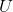be the set of all positive integers.

Letbe the set of all multiples of 3; letbe the set of all multiples of 7; letbe the set of all perfect square integers. Which of the following statements is true of 243?

Note: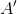means "the complement of", etc.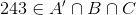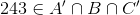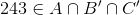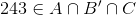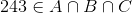Explanation: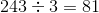, so 243 is divisible by 3.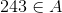.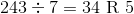, so 243 is not divisible by 7.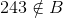- that is,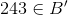.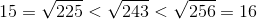, 243 is not a perfect square integer.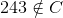- that is,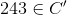.

Since 243 is an element of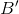, and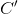, it is an element of their intersection. The correct choice is that### Example Question #7 : How To Find The Square Of An Integer

Consider the inequality: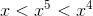Which of the following could be a value of?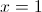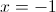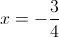There is no possible value for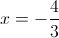Explanation:

Notice how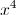is the greatest value. This often means thatis negative as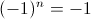when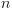is odd and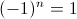whenis even.

Let us examine the first choice,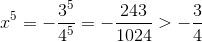This can only be true of a negative value that lies between zero and one.

### Example Question #8 : How To Find The Square Of An Integer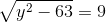In the equation above, ifis a positive integer, what is the value of?Explanation:

Begin by squaring both sides of the equation: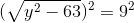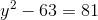Now solve for y: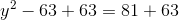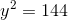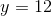Note thatmust be positive as defined in the original question. In this case,must be 12.